# Least Common Multiples and Greatest Common Factors Least

• Slides: 13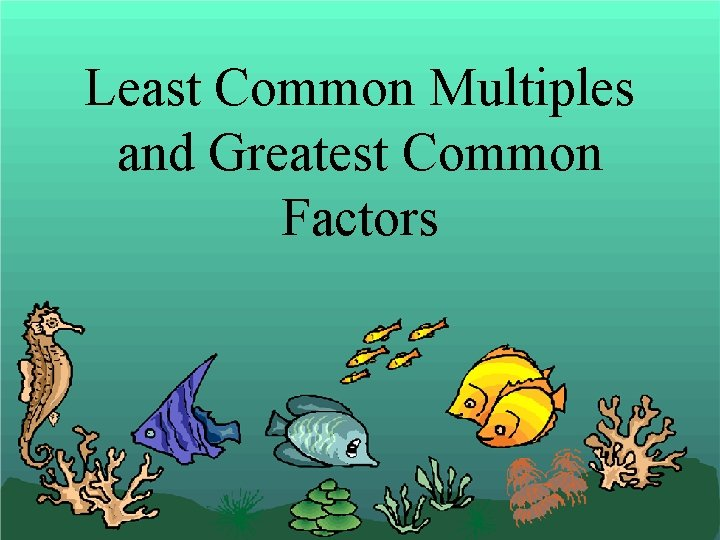Least Common Multiples and Greatest Common Factors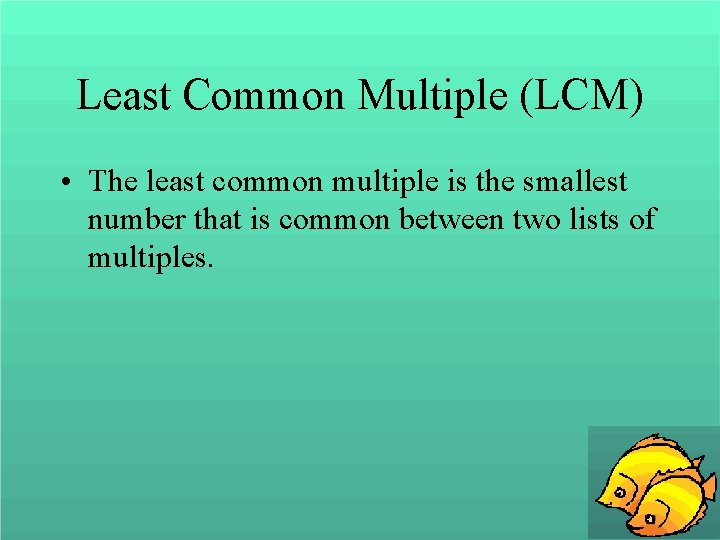Least Common Multiple (LCM) • The least common multiple is the smallest number that is common between two lists of multiples.EXAMPLE: Find the LCM of 12 and 18 The multiples of 12: The multiples of 18: • 12 x 1 = 12 • 18 x 1 = 18 • 12 x 2 =24 • 18 x 2 = 36 • 12 x 3 = 36 • 18 x 3 = 54 • 12 x 4 = 48 • 18 x 4 = 72 • 12 x 5 =60 • 18 x 5 = 9012, 24, 36, 48, 60 18, 36, 54, 72, 90 The first number you see in both lists is 36. The least common multiple of 12 and 18 is 36.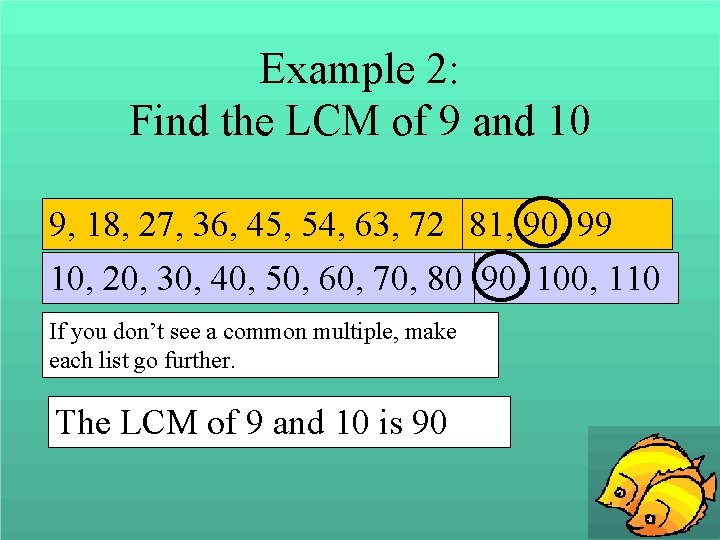Example 2: Find the LCM of 9 and 10 9, 18, 27, 36, 45, 54, 63, 72 81, 90, 99 10, 20, 30, 40, 50, 60, 70, 80 90, 100, 110 If you don’t see a common multiple, make each list go further. The LCM of 9 and 10 is 90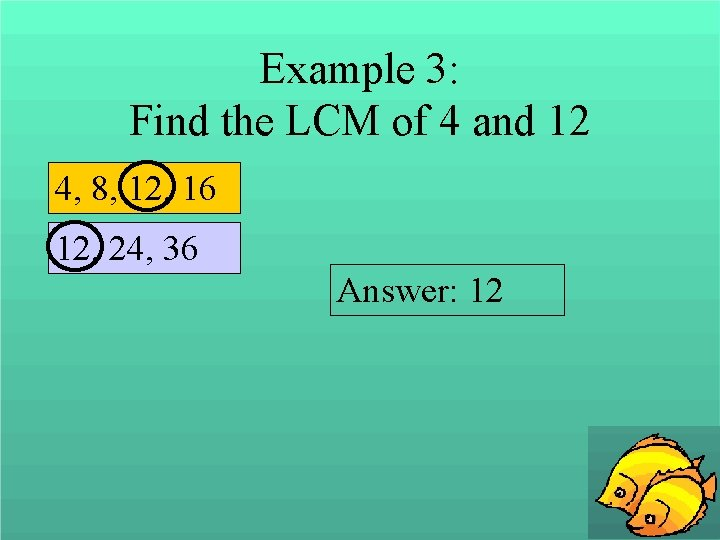Example 3: Find the LCM of 4 and 12 4, 8, 12, 16 12, 24, 36 Answer: 12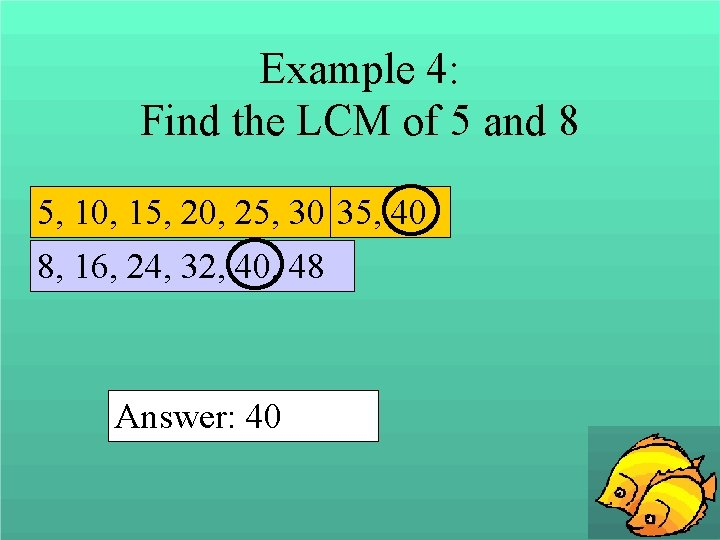Example 4: Find the LCM of 5 and 8 5, 10, 15, 20, 25, 30 35, 40 8, 16, 24, 32, 40, 48 Answer: 40Example 5: Find the LCM of 6 and 20 6, 12, 18, 24, 30, 36 42, 48, 54, 60 20, 40, 60, 80, 100, 120 Answer: 60Greatest Common Factor (GCF) • The greatest common factor is the largest factor that two numbers share. • Let’s find the GCF of 12 and 42. First, we need to make a list of factors for each number.12 1 x 12 42 1 x 42 Factors of 12: 2 x 21 2 x 6 3 x 14 3 x 4 1, 2, 3, 4, 6, 12 4 x ? ? 4 x 3 Factors of 42: 5 x ? ? 1, 2, 3, 6, 7, 14, 21, 6 x 7 7 x 6 42 Common Factors: 1, 2, 3, 6 Greatest Common Factor: 6What is the GCF of 18 and 27? 18 1 x 18 2 x 9 3 x 6 4 x? 5 x? 6 x 3 Factors of 18: 1, 2, 3, 6, 9, 18 Factors of 27: 1, 3, 9, 27 Common Factors: 1, 3, 9 GCF: 9 27 1 x 27 2 x? 3 x 9 4 x? 5 x? 6 x? 7 x? 8 x? 9 x 3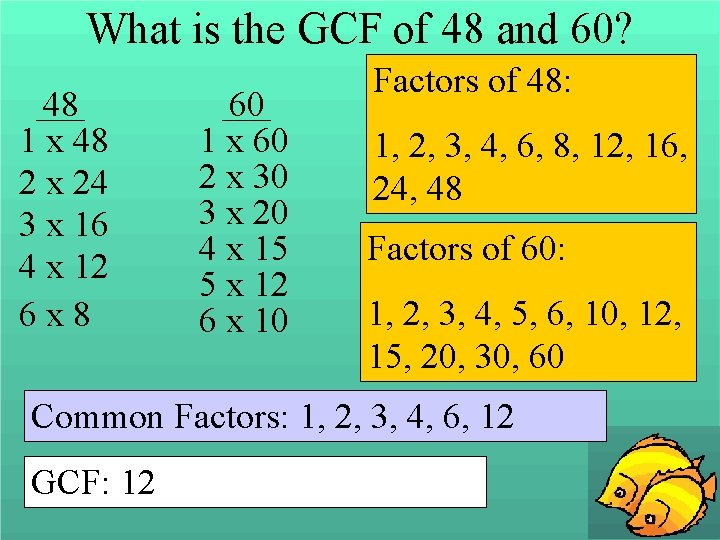What is the GCF of 48 and 60? 48 1 x 48 2 x 24 3 x 16 4 x 12 6 x 8 60 1 x 60 2 x 30 3 x 20 4 x 15 5 x 12 6 x 10 Factors of 48: 1, 2, 3, 4, 6, 8, 12, 16, 24, 48 Factors of 60: 1, 2, 3, 4, 5, 6, 10, 12, 15, 20, 30, 60 Common Factors: 1, 2, 3, 4, 6, 12 GCF: 12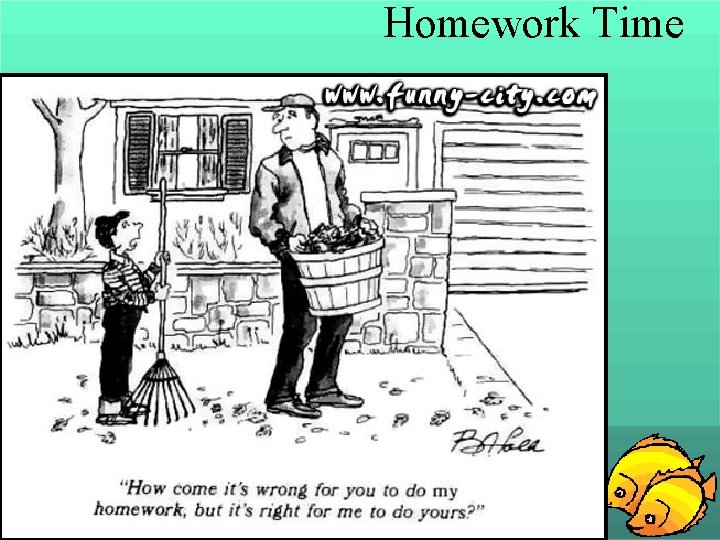Homework Time